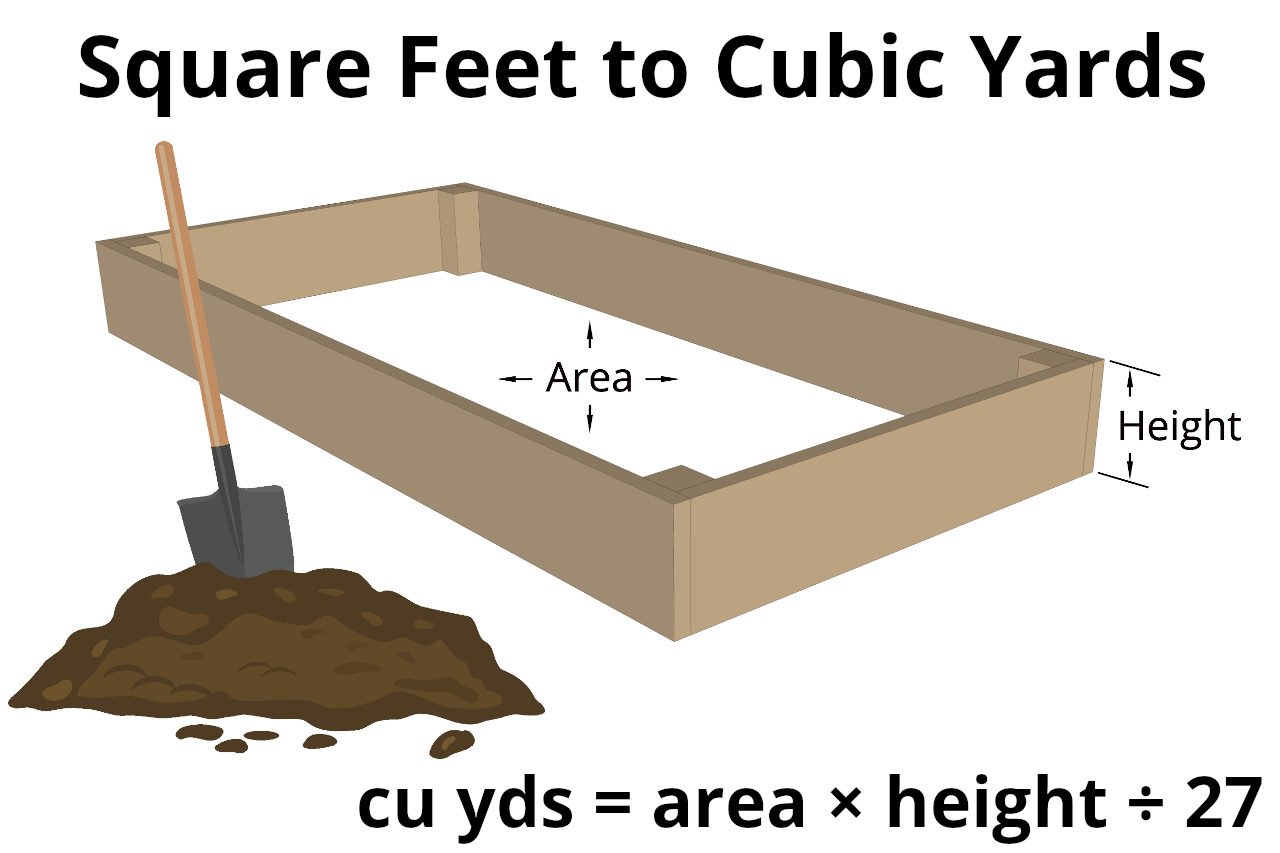# Square Feet to Cubic Yards Calculator

Convert square feet to cubic feet or vice-versa using the calculator below. Read on to learn how we calculated the result.

Cubic Yards

Cubic Feet

## How to Convert Square Feet to Cubic Yards

To convert square feet to cubic yards, you’ll also need the height or depth. This is because these are different units of measure, so to convert between them, you need the height.

Square feet measure the area of a space, while cubic yards measure its volume.

To convert, you can use a formula.### Square Feet to Cubic Yards Formula

The formula to convert is:

cu yds = sq ft × heightft ÷ 27

Thus, the volume of a space in cubic yards is equal to the area in square feet times the height in feet, divided by 27. Essentially, you’re calculating the volume in cubic feet, then by dividing by 27, you’re converting the result to cubic yards.

If you don’t know the square footage, you can always use our square footage calculator, or just use this formula:

cu yds = (lengthft × widthft × heightft) ÷ 27

### Steps to Convert

Now that you know the conversion formula, follow these steps to convert square feet to cubic yards.

Step one: calculate the square footage of the space by multiplying the length and width.

Step two: calculate the cubic footage of the space by multiplying the square footage by the height or depth in feet.

Step three: calculate the cubic yardage of the space by dividing the cubic footage by 27. You can also use a cubic yards calculator for this.

## How to Convert Cubic Yards to Square Feet

You can convert cubic yards back to square feet by reversing the steps above. Of course, you’ll need to know the conversion formula.

### Cubic Yards to Square Feet Formula

Use this formula to convert cubic yards to square feet:

sq ft = (cu yds × 27) ÷ heightft

The area in square feet is equal to the volume in cubic yards times 27, divided by the height in feet.

You might also be interested in our square feet to cubic feet calculator.